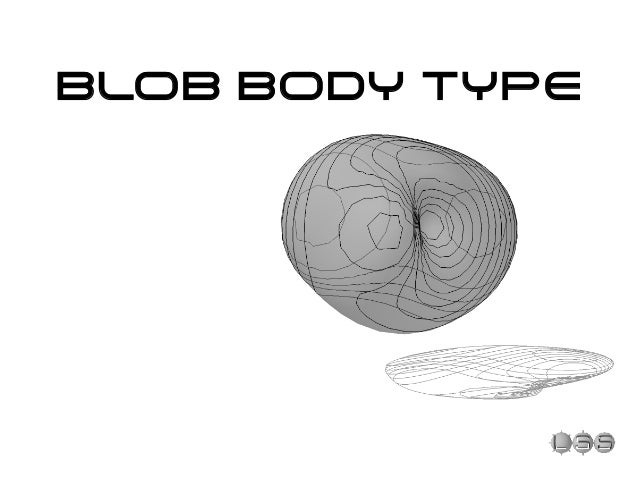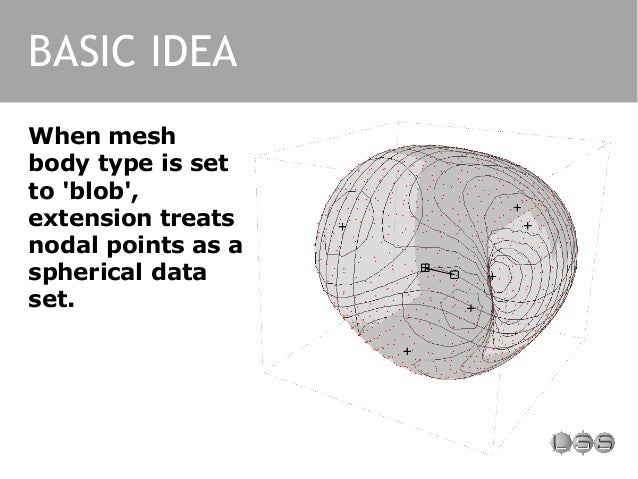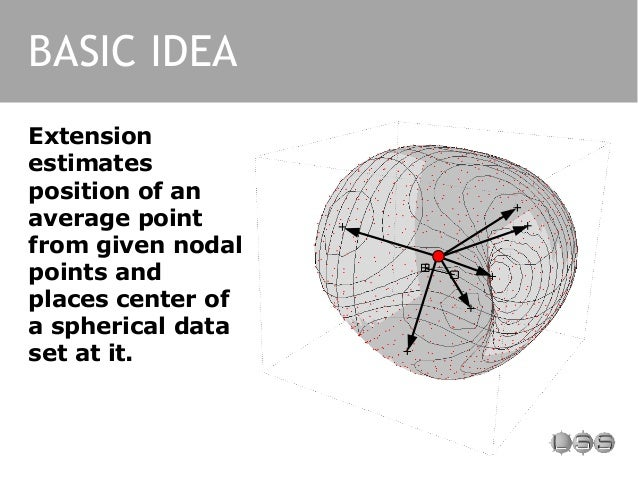Successfully reported this slideshow.Upcoming SlideShare
×

ofUpcoming SlideShare
Lss arch 1.2
Next

0 Likes

Share

# Blob Body Type Explanations

Learn more about new mesh body type introduced in version 1.3 of LSS Arch extension for SketchUp.

See all

### Related Audiobooks

#### Free with a 30 day trial from Scribd

See all
• Be the first to like this

### Blob Body Type Explanations

1. 1. BLOB BODY TYPE
2. 2. BASIC IDEA When mesh body type is set to 'blob', extension treats nodal points as a spherical data set.
3. 3. Extension estimates position of an average point from given nodal points and places center of a spherical data set at it. BASIC IDEA
4. 4. During interpolation length of radius vectors to unknown points are calculated according to selected interpolation algorithm. BASIC IDEA
5. 5. Inverse Distance Weighting IDW ALGORITHM
6. 6. IDW ALGORITHM Inverse Angle Weighting (IAW) would be a more accurate name because algorithm actually uses angle to measure 'amount of proximity'
7. 7. 'Average' and 'minimize' algorithms ITERATIVE ALGORITHMS
8. 8. First stage is a preliminary calculation, which sets the same length to a radius vector to a point being calculated as a length of a vector to a closest* known nodal point. ITERATIVE ALGORITHMS * - «closest» in terms of spherical coordinate system here
9. 9. The second stage is an iterative smoothing itself: points within a cell size angle from known points stay where they are, others gradually move along their radius vectors. ITERATIVE ALGORITHMS
10. 10. So the process is similar to the one that takes place during conventional 'terrain-like' mesh processing. It is just adapted a bit for spherical coordinate system. ITERATIVE ALGORITHMS
11. 11. TREND AND PREDICT Mapped surface
12. 12. Adaptation of these algorithms for spherical coordinate system is a bit more tricky. Extension maps given points to Cartesian coordinate system at first... TREND AND PREDICT
13. 13. TREND AND PREDICT ...then processes mapped set as usual.
14. 14. IDW VS PREDICT Radius vector to each given nodal point is normal to a surface tangent plane at this point, when algorithm is set to IDW. So known points are local extrema. IDW
15. 15. IDW VS PREDICT The idea of 'predict' algorithm is to estimate surface tangent planes at given nodal points. 'Predict'
16. 16. IDW VS PREDICT Known points are not necessarily local extrema in case when 'predict' algorithm is chosen so result of interpolation can be more convincing.

Total views

3,723

On Slideshare

0

From embeds

0

Number of embeds

2,783

4

Shares

0